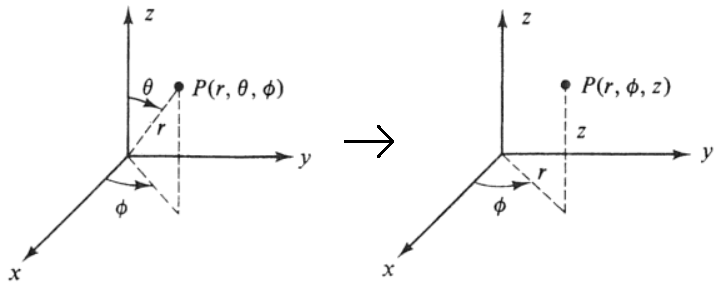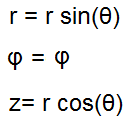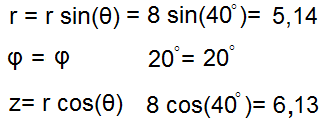﻿ Spherical to Cylindrical Coordinates Calculator ﻿# Spherical to Cylindrical Coordinates Calculator### Spherical to Cylindrical Coordinate System Diagramr: θ: φ: ° rad

This cylindrical coordinates converter/calculator converts the spherical coordinates of a unit to its equivalent value in cylindrical coordinates, according to the formulas shown above.

Spherical coordinates are depicted by 3 values, (r, θ, φ). When converted into cylindrical coordinates, the new values will be depicted as (r, φ, z).

To use this calculator, a user just enters in the (r, θ, φ) values of the spherical coordinates and then clicks 'Calculate', and the cylindrical coordinates will be automatically computed and shown below.

By default, the calculator will compute the result in degrees. However, by using the drop-down menu, the option can changed to radians, so that the result will be computed in radians.

Example Calculation

Convert the spherical coordinates (8, 40°, 20°) into its equivalent cylindricals coordinates.This answer is calculated in degrees mode.

Related Resources

﻿